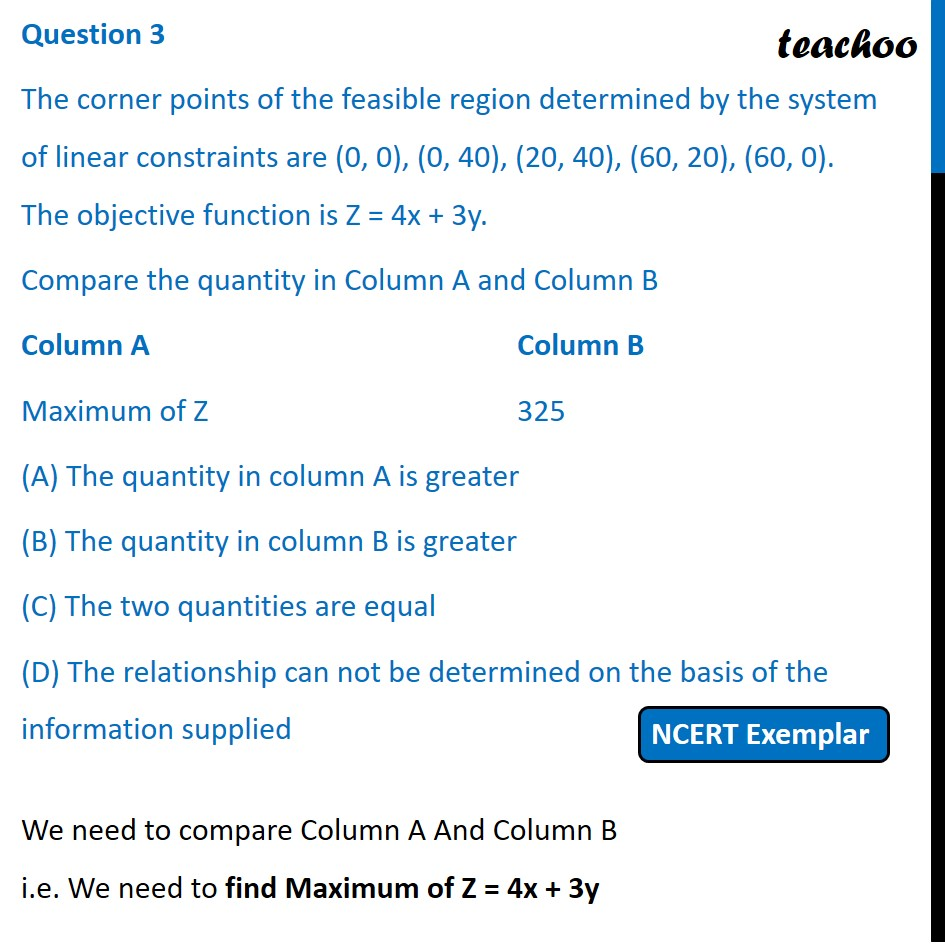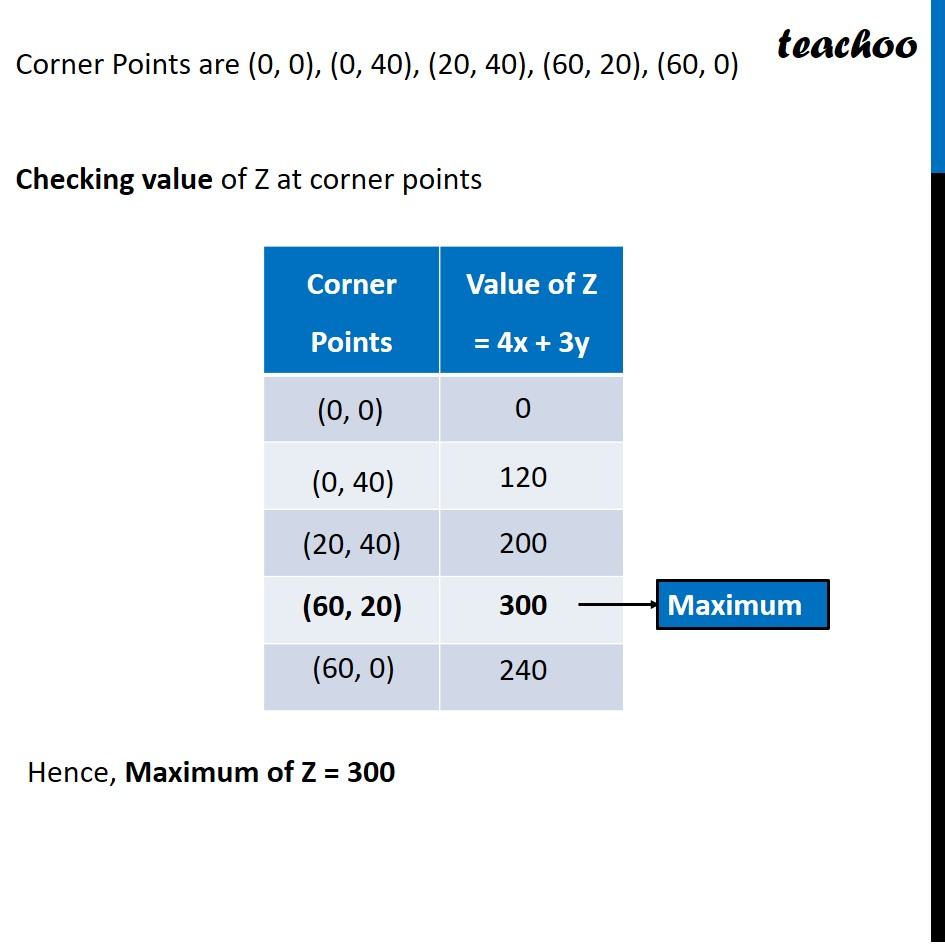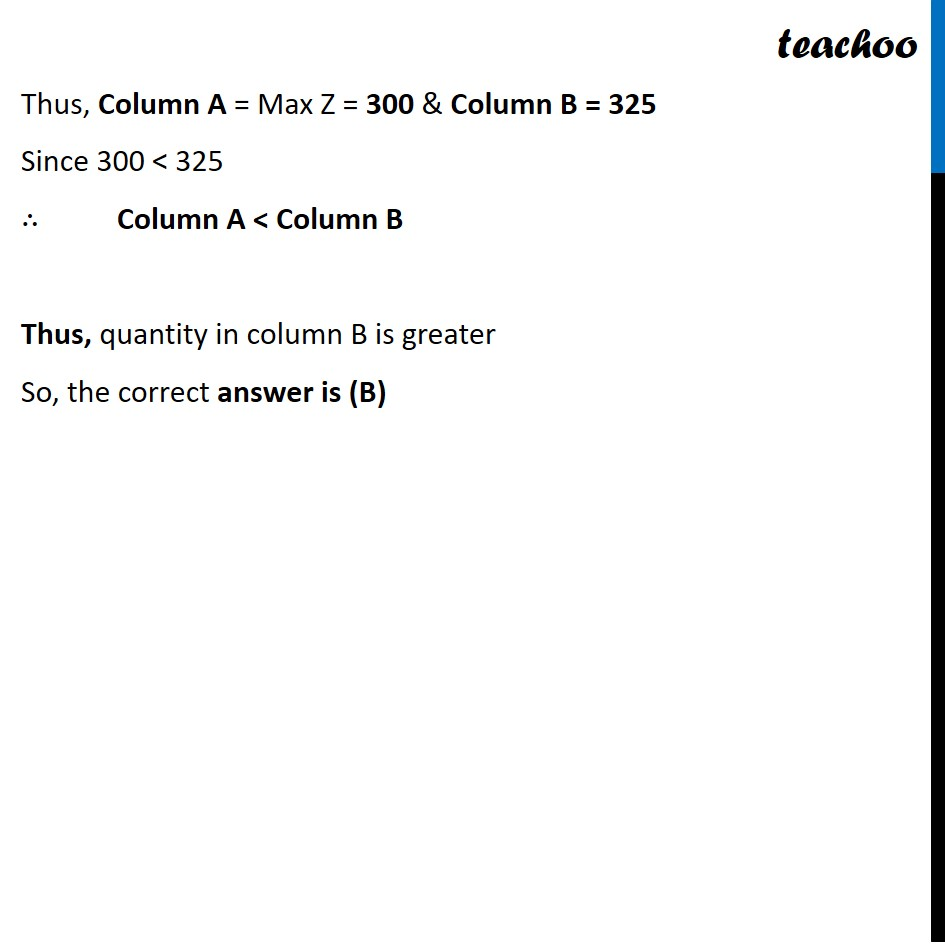NCERT Exemplar - MCQs

Chapter 12 Class 12 Linear Programming
Serial order wise

## (D) The relationship can not be determined on the basis of the information supplied

This question is similar to Question 38 Choice 2 - Sample Paper 2021 Class 12 - Linear ProgrammingLearn in your speed, with individual attention - Teachoo Maths 1-on-1 Class

### Transcript

Question 3 The corner points of the feasible region determined by the system of linear constraints are (0, 0), (0, 40), (20, 40), (60, 20), (60, 0). The objective function is Z = 4x + 3y. Compare the quantity in Column A and Column B Column A Column B Maximum of Z 325 (A) The quantity in column A is greater (B) The quantity in column B is greater (C) The two quantities are equal (D) The relationship can not be determined on the basis of the information supplied We need to compare Column A And Column B i.e. We need to find Maximum of Z = 4x + 3y Corner Points are (0, 0), (0, 40), (20, 40), (60, 20), (60, 0) Checking value of Z at corner points Hence, Maximum of Z = 300 Thus, Column A = Max Z = 300 & Column B = 325 Since 300 < 325 ∴ Column A < Column B Thus, quantity in column B is greater So, the correct answer is (B)